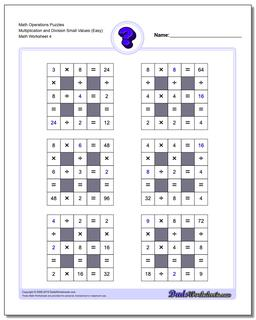# Math Worksheets: Number Grid Puzzles: Number Grid Puzzles: Math Operations Puzzles Multiplication and Division Small Values (Easy) (Fourth Worksheet)## Math Operations Puzzles Multiplication and Division Small Values (Easy) (Fourth Worksheet)

PropertyValue
DescriptionMath Operations Puzzles Multiplication and Division Small Values (Easy): Math puzzle worksheets that require students to fill in missing numbers with values up Small Numbers. (Fourth Worksheet)
Resource TypeWorksheet